# Connected scatter plot in R

## Connected scatter plot

A connected scatter plot represents the relationship between two variables, generally through the time. You can create this type of chart in base R with the `plot` function, setting `type = "b"`.

``````# Data
x <- c(1, 2, 3, 4, 5, 4, 7, 8, 9)
y <- c(12, 16, 14, 18, 16, 13, 15, 20, 22)

# Connected scatter plot
plot(x, y, type = "b",
xlab = "Var 1", ylab = "Var 2")``````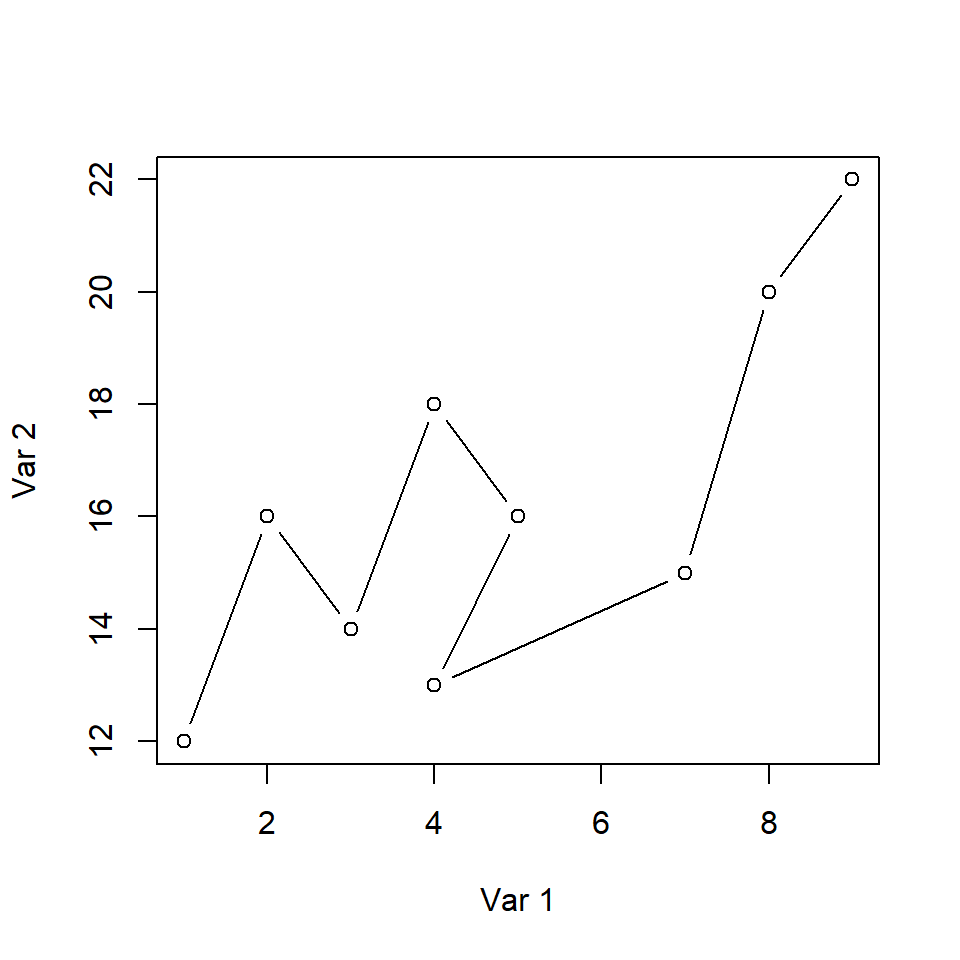Symbol

The symbol used by default when `type = "b"` can be modified making use of the `pch` argument.

``````# Data
x <- c(1, 2, 3, 4, 5, 4, 7, 8, 9)
y <- c(12, 16, 14, 18, 16, 13, 15, 20, 22)

plot(x, y,
type = "b", pch = 18,
xlab = "Var 1", ylab = "Var 2")``````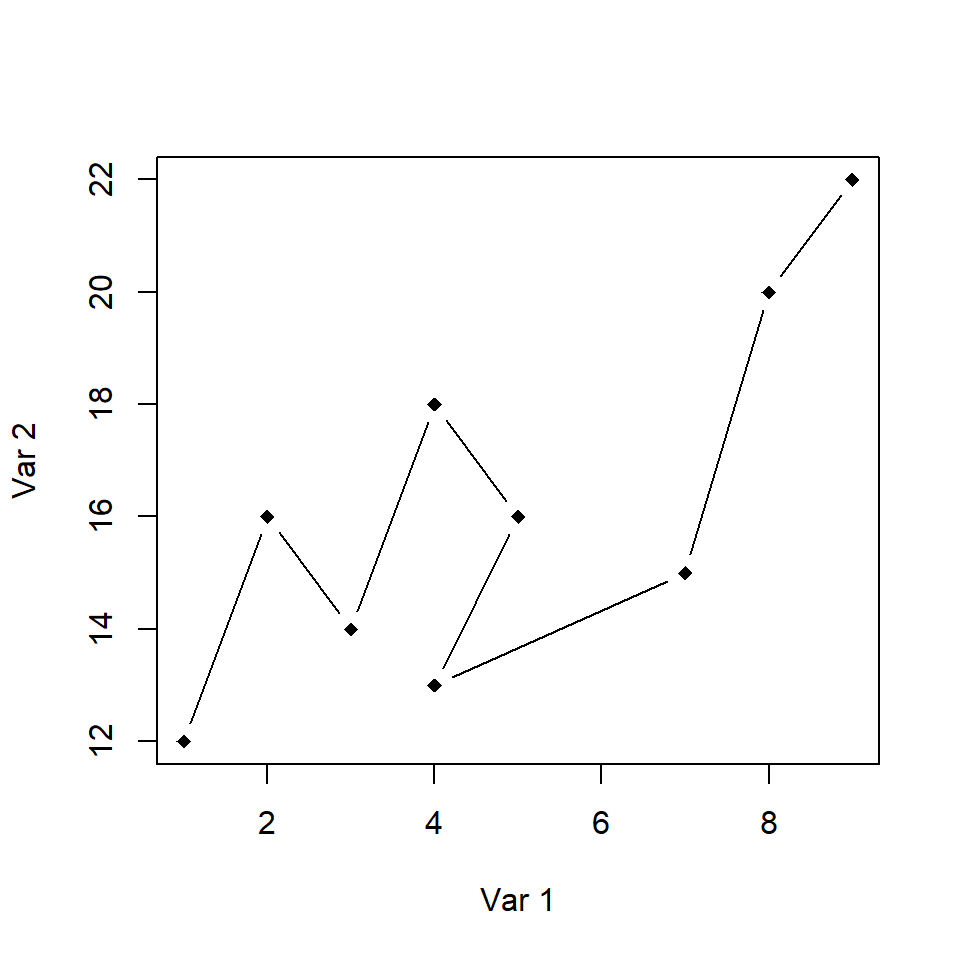Color and points

You can change the color of the lines and points. However, the color of the points and the lines will be the same. You can override the points using the `points` function.

``````# Data
x <- c(1, 2, 3, 4, 5, 4, 7, 8, 9)
y <- c(12, 16, 14, 18, 16, 13, 15, 20, 22)

# Custom points and color
plot(x, y, type = "b",
pch = 19, col = 4,
xlab = "Var 1", ylab = "Var 2")
points(x, y, pch = 19)``````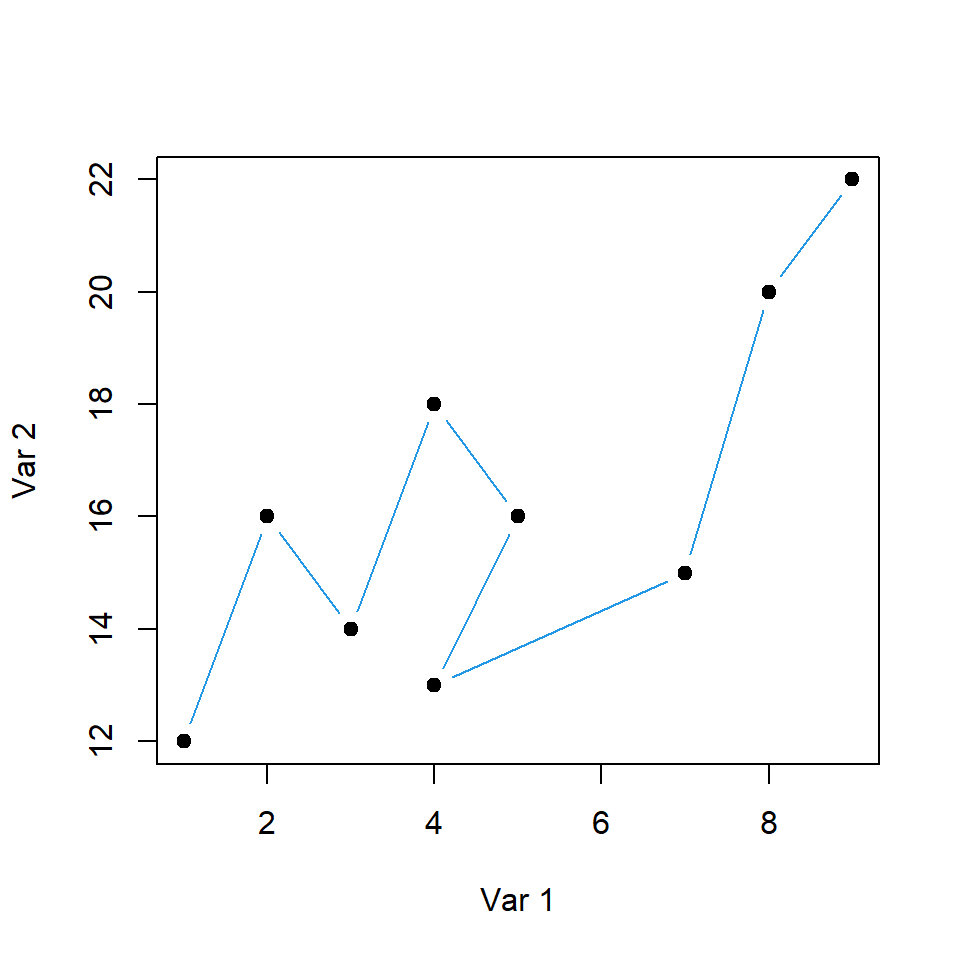You can label the points with the `text` function. This can be useful, for instance, to label the date for each pair of observations. Note that we fine tuned the position of the labels for better readability.

``````# Data
x <- c(1, 2, 3, 4, 5, 4, 7, 8, 9)
y <- c(12, 16, 14, 18, 16, 13, 15, 20, 22)
labels <- 2013:2021

# Connected scatter plot with text
plot(x, y, type = "b",
xlim = c(1, 10), ylim = c(12, 23),
pch = 19, col = 4,
xlab = "Var 1", ylab = "Var 2")
text(x + 0.7, y + 0.4, labels)``````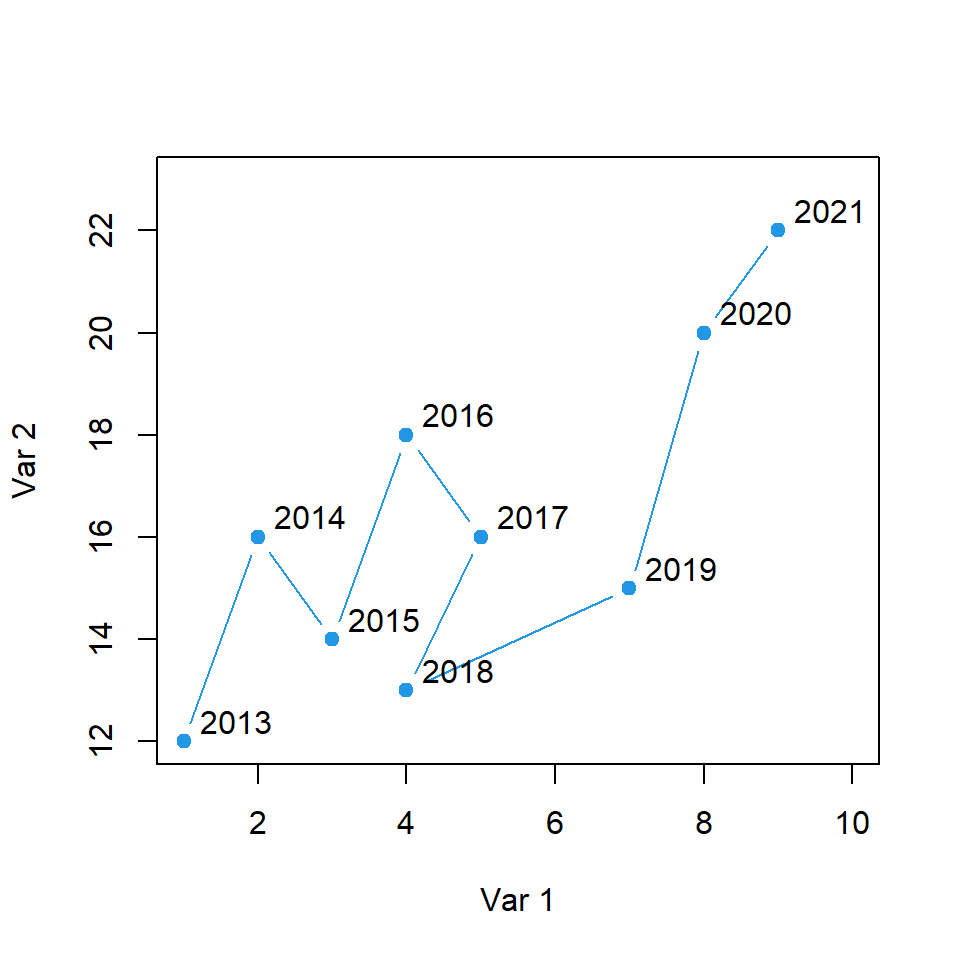## Connected scatter plot with arrows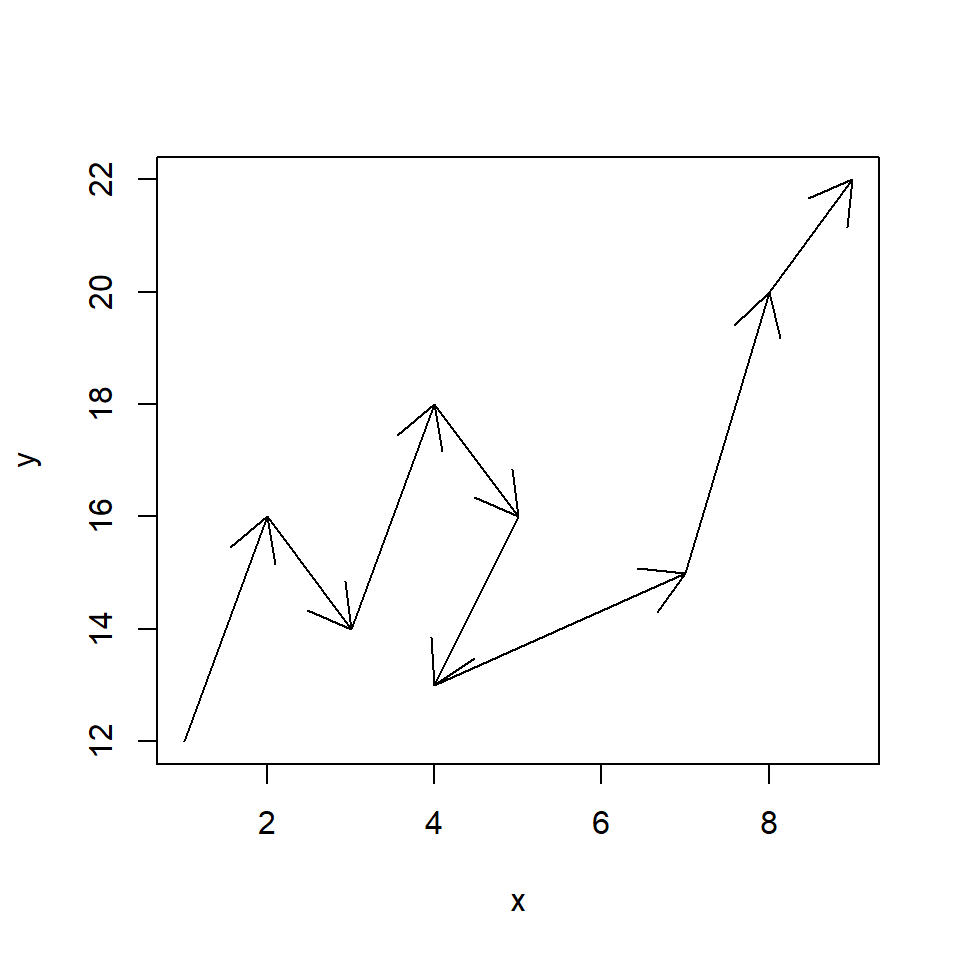Connected scatter plot with arrows

Other possibility is to use arrows to draw a path between the observations of the variables. For that purpose you can use the following function.

``````conArrows <- function(x, y, ...) {
plot(x, y, pch = "", ...)
invisible(sapply(1:length(x),
function(i) arrows(x[i], y[i],
x[i + 1], y[i + 1], ...)))
}

# Data
x <- c(1, 2, 3, 4, 5, 4, 7, 8, 9)
y <- c(12, 16, 14, 18, 16, 13, 15, 20, 22)

# Connected scatter plot with arrows
conArrows(x, y)``````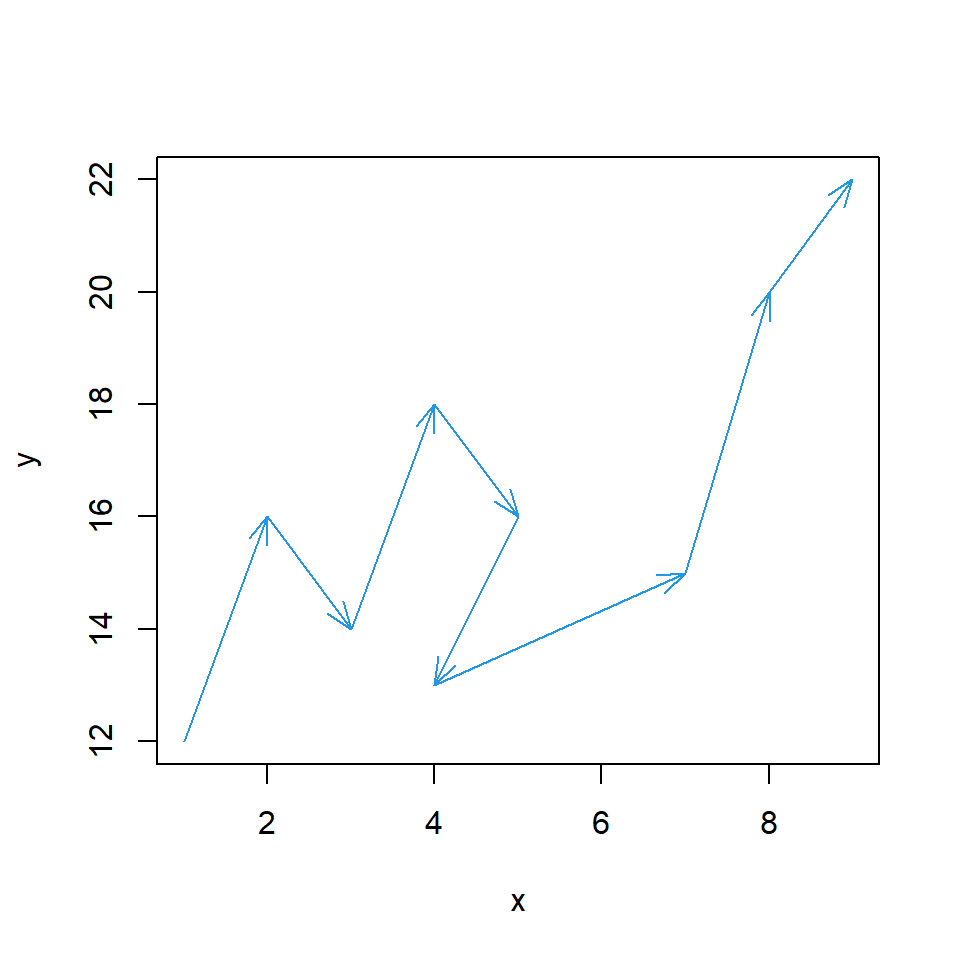Customization

You can pass arguments to the function to customize the output, as shown in the example below.

``````conArrows <- function(x, y, ...) {
plot(x, y, pch = "", ...)
invisible(sapply(1:length(x),
function(i) arrows(x[i], y[i],
x[i + 1], y[i + 1], ...)))
}

# Data
x <- c(1, 2, 3, 4, 5, 4, 7, 8, 9)
y <- c(12, 16, 14, 18, 16, 13, 15, 20, 22)

# Connected scatter plot with arrows
conArrows(x, y, angle = 20, length = 0.15, col = 4)``````